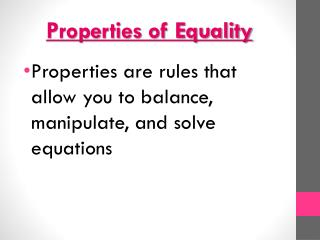# Properties of Equality - PowerPoint PPT PresentationDownload PresentationProperties of Equality

Properties of EqualityDownload Presentation## Properties of Equality

- - - - - - - - - - - - - - - - - - - - - - - - - - - E N D - - - - - - - - - - - - - - - - - - - - - - - - - - -
##### Presentation Transcript

1. Properties of Equality • Properties are rules that allow you to balance, manipulate, and solve equations

2. Addition Property of Equality • Adding the same number to both sides of an equation does not change the equality of the equation. • If a = b, then a + c = b + c. • Ex: x=y, so x+2=y+2

3. Subtraction Property of Equality • Subtracting the same number to both sides of an equation does not change the equality of the equation. • If a = b, then a – c = b – c. • Ex: x = y, so x – 4 = y – 4

4. Multiplication Property of Equality • Multiplying both sides of the equation by the same number, other than 0, does not change the equality of the equation. • If a = b, then ac = bc. • Ex: x = y, so 3x = 3y

5. Division Property of Equality • Dividing both sides of the equation by the same number, other than 0, does not change the equality of the equation. • If a = b, then a/c = b/c. • Ex: x = y, so x/7 = y/7

6. Reflexive Property of Equality • A number is equal to itself. (Think mirror) • a = a • Ex: 4 = 4

7. Symmetric Property of Equality • If numbers are equal, they will still be equal if the order is changed. • If a = b, then b = a. • Ex: x = 4, then 4 = x

8. Transitive Property of Equality • If numbers are equal to the same number, then they are equal to each other. • If a = b and b = c, then a = c. • Ex: If x = 8 and y = 8, then x = y

9. Substitution Property of Equality • If numbers are equal, then substituting one in for the another does not change the equality of the equation. • If a = b, then b may be substituted for a in any expression containing a. • Ex: x = 5, then y = x + 6 is the same as y = 5 + 6.

10. Other Properties

11. Commutative Property • Changing the order of addition or multiplication does not matter. • “Commutative” comes from “commute” or “move around”, so the Commutative Property is the one that refers to moving stuff around.

12. Commutative Property • Addition: a + b = b + a • Ex: 1 + 9 = 9 + 1

13. Commutative Property • Multiplication: a ∙ b = b ∙ a • Ex: 8 ∙ 6 = 6 ∙ 8

14. Associative Property • The change in grouping of three or more terms/factors does not change their sum or product. • “Associative” comes from “associate” or “group”, so the Associative Property is the one that refers to grouping.

15. Associative Property • Addition: a + (b + c) = (a + b) + c • Ex: 1 + (7 + 9) = (1 + 7) + 9

16. Associative Property • Multiplication: a ∙ (b∙ c) = (a ∙ b) ∙ c • Ex: 8 ∙ (3 ∙ 6) = (8 ∙ 3) ∙ 6

17. Distributive Property • The product of a number and a sum is equal to the sum of the individual products of terms.

18. Distributive Property • a ∙ (b + c) = a ∙ b + a ∙ c • Ex: 5 ∙ (x + 6) = 5 ∙ x + 5∙ 6

19. Additive Identity Property • The sum of any number and zero is always the original number. • Adding nothing does not change the original number. • a + 0 = a • Ex: 4 + 0 = 4

20. Multiplicative Identity Property • The product of any number and one is always the original number. • Multiplying by one does not change the original number. • a ∙1 = a • Ex: 2 ∙ 1 = 2

21. Additive Inverse Property • The sum of a number and its inverse (or opposite) is equal to zero. • a + (-a) = 0 • Ex: 2 + (-2) = 0

22. Multiplicative Inverse Property • The product of any number and its reciprocal is equal to 1. • Ex:

23. Multiplicative Property of Zero • The product of any number and zero is always zero. • a ∙ 0 = 0 • Ex: 298 ∙ 0 = 0